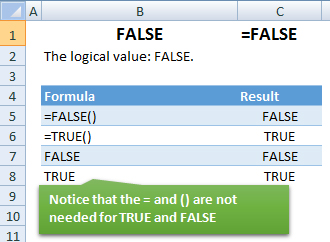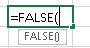### FALSE Function Examples – Excel & Google Sheets

This Excel Tutorial demonstrates how to use the Excel FALSE Function in Excel to return the logical value FALSE, with formula examples.

## FALSE Function Description:

The FALSE Function The logical value: FALSE.

## Formula Examples:AutoMacro - VBA Code Generator

## Syntax and Arguments:

The Syntax for the FALSE Formula is:

`=FALSE`

## Additional Notes

To use the logical values TRUE or FALSE you can simply type “true” or “false”.

Return to the List of all Functions in Excel

## How to use the FALSE Function in Excel:

To use the AND Excel Worksheet Function, type the following into a cell:
`=AND(`
After entering it in the cell, notice how the AND formula inputs appear below the cell:You will need to enter these inputs into the function. The function inputs are covered in more detail in the next section. However, if you ever need more help with the function, after typing “=FALSE(” into a cell, without leaving the cell, use the shortcut CTRL + A (A for Arguments) to open the “Insert Function Dialog Box” for detailed instructions:

For more information about the FALSE Formula visit the
Microsoft Website.

## Excel Practice Worksheet

Practice Excel functions and formulas with our 100% free practice worksheets!

• Automatically Graded Exercises
• Learn Excel, Inside Excel!